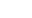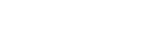How Cheenta works to ensure student success?
Explore the Back-Story

# Calculating Average Speed | Tomato Problem 3This is a problem number 3 from TOMATO based on Calculating Average Speed.

Problem: Calculating Average Speed.

A boy walks from his home to school at 6 kmph. He walks back at 2 kmph. His average speed, in kmph is
(A) 3; (B) 4; (C) 5; (D);

Discussion:

Suppose the distance from home to school is t km.
Time taken for home to school journey:Time taken for school to home journey:Hence average speed =There fore average speed is 3 kmph.

Note that it is the harmonic mean of the two given speeds instead of arithmetic mean.

## Chatuspathi:

• What is this topic: Elementary Arithmetic
• What are some of the associated concepst: Harmonic Mean
• Where can learn these topics: Cheenta I.S.I. & C.M.I. course, discusses these topics in the ‘Number Theory’ module.
• Book Suggestion: Arithmetic by K.C. Nag

This is a problem number 3 from TOMATO based on Calculating Average Speed.

Problem: Calculating Average Speed.

A boy walks from his home to school at 6 kmph. He walks back at 2 kmph. His average speed, in kmph is
(A) 3; (B) 4; (C) 5; (D);

Discussion:

Suppose the distance from home to school is t km.
Time taken for home to school journey:Time taken for school to home journey:Hence average speed =There fore average speed is 3 kmph.

Note that it is the harmonic mean of the two given speeds instead of arithmetic mean.

## Chatuspathi:

• What is this topic: Elementary Arithmetic
• What are some of the associated concepst: Harmonic Mean
• Where can learn these topics: Cheenta I.S.I. & C.M.I. course, discusses these topics in the ‘Number Theory’ module.
• Book Suggestion: Arithmetic by K.C. Nag

This site uses Akismet to reduce spam. Learn how your comment data is processed.

### Knowledge Partner